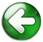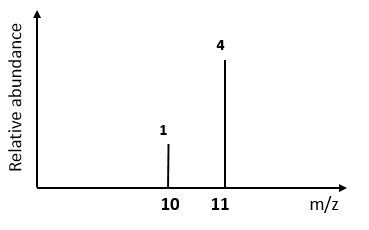Mass spectroscopy and Ar calculations## 1)   A sample of sulphur has three isotopes: sulphur-32, sulphur-33 and sulphur-34. Calculate the relative atomic mass from the data below:  Give your answer to the appropriate number of decimals places.

 percentage abundance 95.02 0.76 4.22 Isotopic mass 32 33 34

RAM = S  (Abundance x m/z)

Total  Abundance

RAM  =  (95.02 x 32)  +  (0.76 x 33)  +  (4.22 x 34)

(95.02  +  0.76  +  4.22)

RAM  =  32.09    (2dp as question is to 2dp)

2)  Boron has 2 isotopes and the mass spectrum is shown below.  Calculate its relative atomic mass:RAM  =  (1 x 10)  +  (4 x 11)                                                  (1  +  4)                           RAM  =  10.8

3)   Lithium has two naturally occurring isotopes 6Li and 7Li. Calculate the percentage abundance of each isotopes given that the Ar of Lithium is 6.9.  Show your working.

6.9 is 9/10 th's between 6Li and 7Li

So 90 % must be 7Li so 10 % must be 6Li

OR:

RAM = S  (Abundance x m/z)

Total  Abundance

6.9  =  (A x 6)  +  ((100-A) x 7))               Where A is the Abundance of 6Li